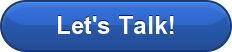Have questions? Need Help?
757.723.6531 | 800.950.2468

# Teledyne Hastings Instruments Blog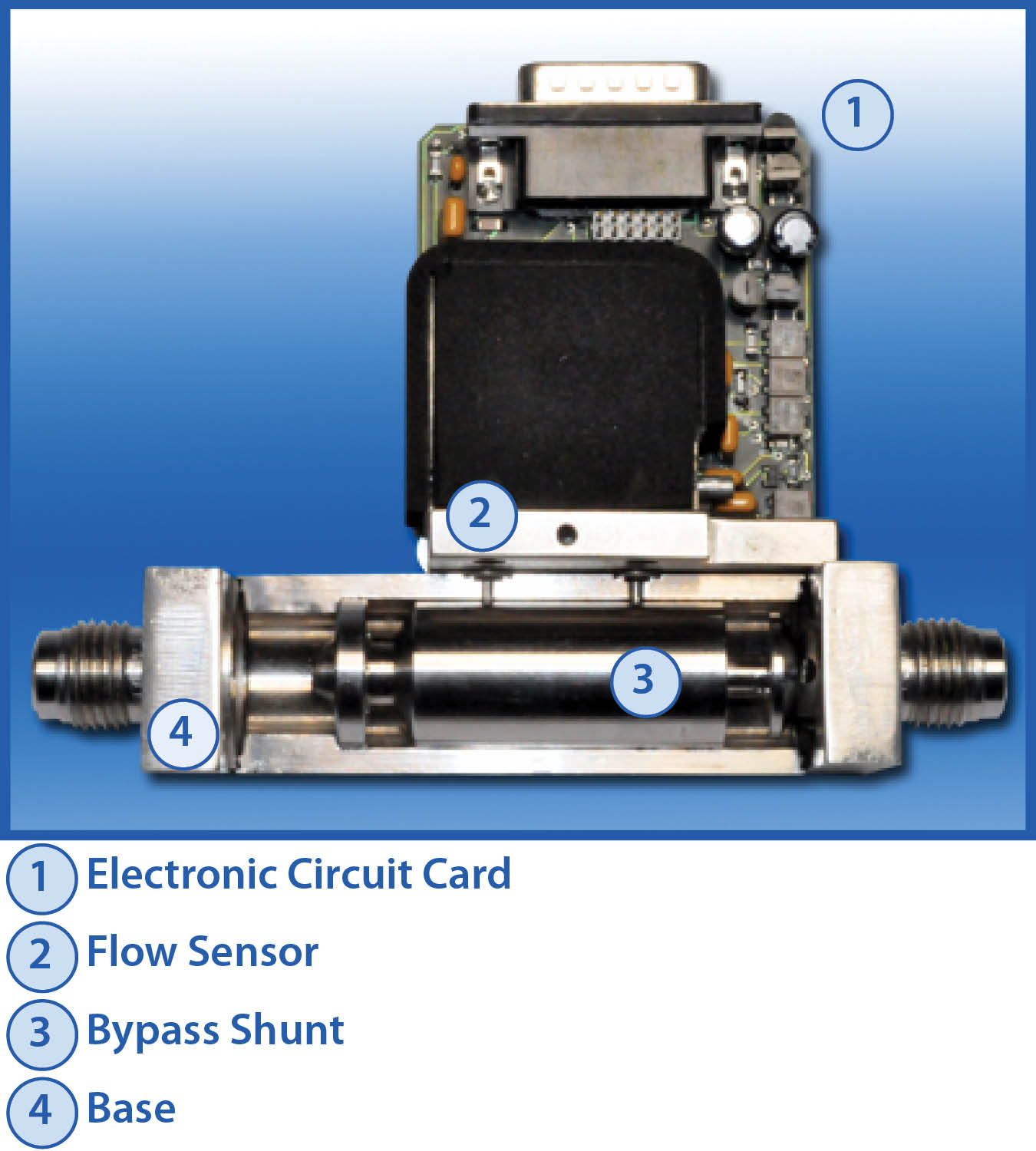This is part 1 of a 2-part blog on the Thermal Mass Flow Meter.  In Part 1 we will explain the desired characteristics of a mass flow meter (and its sensor). Part 2 will discuss the operation of the Teledyne Hastings 300 Series flow meters (Patent #6,125,695) and how the 300 Series thermal mass flow sensor meets each of the desired characteristics described below.

## What is a thermal mass flow meter:

• Electronic Circuit Card

• Flow Sensor

• Bypass Shunt

• Base

A cutaway is shown in the image on the right.

A flow meter measures the amount of fluid that passes through the meter. At Teledyne Hastings we design our thermal mass flow meters for dry and clean gases. This is useful for wide array applications that include measuring natural gas or air and biogas measurement.

In a typical mass flow meter, gas enters the meter via an upstream port connected to the process to be measured (by Swagelok®, VCR®    or other fitting).  A majority of the gas passes through the meter’s bypass shunt; however, a certain fraction flows through the meter’s thermal flow sensor.

The meter’s thermal mass flow sensor measures the gas molecular flow that passes through its capillary tube by quantitating thermal energy transfer. The mass flow rate is a function of the gas flow and the specific heat of the gas. The thermal mass flow sensor then provides accurate measurements which can be referenced back to standardized volumetric flow units.  Reference conditions (standard temperature and pressure) are based upon the amount of gas flow, which is determined by the number of gas molecules, using the ideal gas law. The meter’s shunt is selected such that the amount of gas moving through the flow sensor is approximately the same at full-scale flow. After passing through the thermal mass flow sensor, the gas then exits the flow meter via a downstream port.

Thermal Mass Flow Meter Characteristics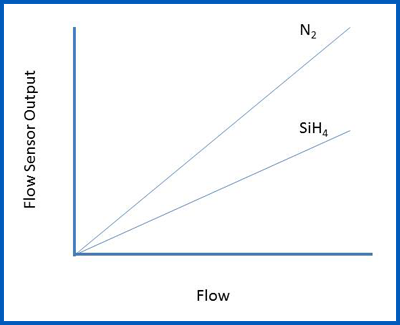Ideally, a thermal mass flow sensor will exhibit the following characteristics:

• Linearity: Linearity means that the sensor’s electronic output is directly proportional to the rate of gas flow that is moving through the sensor (within its range). Linearity of the flow sensor leads to the second attribute: Accuracy.
• Accuracy: Accuracy is dependent on the sensor’s Linearity. An accurate flow sensor provides the benefits of better gas thermal flow measurement, flow control and a thorough understanding of the system’s parameters.
• Fast Response:  Ideally, the flow sensor would respond instantaneously to a change in the mass flow rate. Aside from the obvious benefit of instant real-time oversight of the process flow, fast response becomes critical when the flow meter is coupled with a proportional control valve to create a thermal mass flow controller.
• Low Differential Drop: For a flow sensor to be ideal for leak testing, it should have a low differential pressure drop across the meter.

Typically, a mass flow meter is calibrated using nitrogen gas (or in the case of very large flows, it may be calibrated in air). The output of the flow meter can then be scaled for use with other process gases. This means the flow meter technician can calibrate a flow meter for use with a corrosive process gas, such as silane (SiH4), without having to use that specific type of gas. A linear flow sensor will retain its linear behavior as the gas is switched from the calibration gas (N2) to the process gas.

## How does a thermal mass flowmeter work?

When gas is flowing through the bypass shunt, a small pressure drop is developed which will direct a fraction of the flow through the arced / semi-circular capillary tubing in the flow sensor. On the outside of the capillary tube, there are two resistive wire coils that act as temperature sensors which are tightly wound and in excellent thermal contact with the tube. These two identical windings are referred to as:

• Upstream Heater Coil (1)
• Downstream Heater Coil (2)

Associated with each of the two heated coils is an ambient coil. The ambient coil is in excellent thermal contact with the aluminum ambient block.  Aluminum has a very high thermal conductivity which ensures that both ends of the sensor tube and the two ambient coils (3 and 4) will be at the same temperature.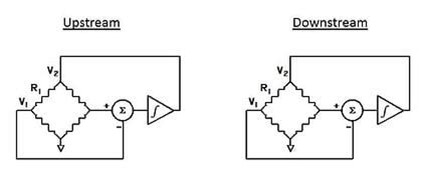Two identical Wheatstone resistance bridges are formed from the two pair of coils (see image on right).

The circuit shown in the image on the right is designed to ensure that the heated sensor (upstream and downstream) are maintained at a constant temperature differential (ΔT) above the corresponding ambient coils.

Next, we calculate the power (W) required to maintain ΔT by:This power will be calculated for both the upstream bridge and the downstream bridge. It can be shown that: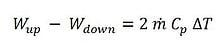So, by maintaining both heaters at the same temperature difference (ΔT) above ambient, the mass flow rate is directly proportioned to the difference in power (W) between the two bridges. For example, when no flow is passing through the capillary sensor tube, the power needed to maintain that temperature difference (ΔT) will be the same (i.e. ṁ = 0)

As gas flow increases in the tube, heat is transferred from the upstream heater to the gas stream.  This will force the upstream circuit to use more power to maintain ΔT. In turn, the gas will transfer heat to the downstream heater which will cause the downstream circuit to use less power to maintain ΔT.

Now, here is the best part: the mass flow rate is directly proportional to the power difference. In other words, Linearity!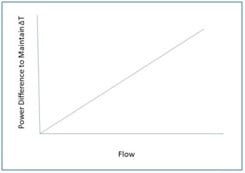## Application

Measuring gas flows has become increasingly critical to many processes and the mass flow meter achieves those results with a high level of accuracy.  Accurate readings must reference standard temperature and pressure (STP) conditions, without having to correct for temperature and pressure using volumetric flow meters. Typically this requires a temperature compensation for the fluid temperature. Using this method is also not a direct mass measurement because the only direct measurement taken is of the fluid temperature. This can be used for heavy gases such as natural gas or gases as light as hydrogen.

Thermal mass flow meters exhibiting low pressure differentials are ideal for measuring flow in leak testing applications and must provide fast response and accurate gas flow readings.

Teledyne Hastings designed its first fast-response flow meter for leak testing applications in the automotive industry. The low-pressure differential and response speed proved to be highly successful. Today, Teledyne Hastings' Thermal Mass Flow Sensors are used globally in a variety of diverse industries and applications.  For more information on Best Practices for Flow Controllers and Thermal Mass Flow Meters download our whitepaper.Be sure to visit our website for additional information on Teledyne Hastings Mass Flow Controllers and Mass Flow Meters

In our next blog, we will discuss the thermal mass flow sensor at the heart of Teledyne 300 Series of mass flow meters. We will also look at how the 300 Series thermal mass flow sensor meets each of the desired characteristics described above.

Tags: Thermal Flow

As I go through the day looking at various mass flow applications, I often notice that it is very easy for users to overlook one of the crucial items required for calculating mass flow. Looking at an application with its established requirements, we often jump right to determining “what flow rate is required?” However, it is important to remember that mass flow applications using volumetric units must reference a standard temperature and pressure. But why is this the case?

When examining liquid flow instruments, we know that liquids are incompressible and thus the amount of a substance present is determined by the volume being used. This leads to a simple calculation using density with the already determined volume to find the mass present in the volume or the volumetric flow.Gases, however, ARE compressible and so the volume is only one factor in determining the amount of material being measured. If we look at the ideal gas law that you may remember from a chemistry class school (PV = nRT), we understand that temperature (T) and pressure (P) must also be considered in the equation. Otherwise it is impossible for us to know “how much” of the substance (n) there is in the space (V) or flowing through the system.

But given all of this information do we actually end up with the mass flow? The actual quantification of this “how much” calculation is expressed in moles (n), which is an extremely large number of molecules of a gas stated as Avogadro’s number, equal to 6.02x1023 (Don’t be scared by this value, though. A mole is a number, just like one dozen is 12, so one mole is 6.02x1023 molecules). Since the number of molecules of a gas and the mass are directly related for each gas type (i.e. molar mass), we are able to calculate the mass of the volume or volumetric flow based on the number of moles present. This is based on the assumption that the measured gas is pure and not contaminated with any other gases.

We’ll look at an example of the difference of STP conditions in a mass flow meter.  Teledyne Hastings Instruments assumes STP of 0°C and 760 Torr, but would prefer the customer to specify their STP conditions for the application. We will use the frequently referenced STP of 20°C and 760 Torr for the second part of this example. Suppose that we are looking to  measure 1 SLM (Standard Liter per Minute) of Nitrogen gas. As I’ve discussed earlier, the 1 SLM must be referenced to an STP value, so we will use our assumed conditions of 0°C and 760 Torr. If we were to change to the second set of conditions, the number of moles present in the flow (Molar Flow Rate) would change, and our mass flow rate would thus change (based on the direct relationship between mass and moles). Our initial mass flow rate of 1 SLM of Nitrogen at 0°C and 760 Torr would now be 1.074 SLM of Nitrogen at 20°C and 760 Torr.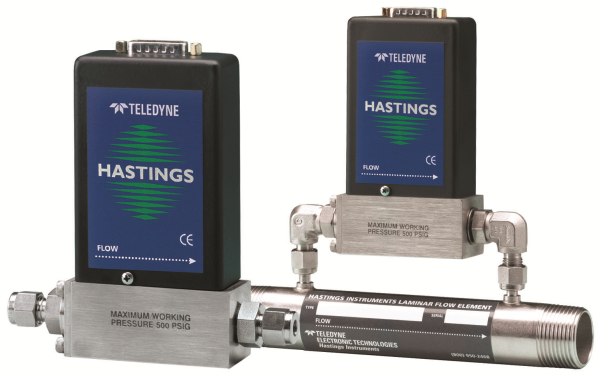An important item to note is that the STP conditions are not actually present during the calibration of mass flow meters and mass flow controllers. Gas conditions are not brought to 0°C and 760 Torr prior to running calibration of equipment. The substance may not even be in gas phase at 0°C. The STP conditions are simply stated to define the standard volumetric flow rates of a substance IF it were an ideal gas at standard conditions.

This is also the reasoning for the addition of the “S” or “Standard” at the start of the stated volumetric flow rate (e.g. Standard Liters per Minute (SLM) or Standard Cubic Centimeters per Minute (SCCM)). We are stating the volumetric flow that would be present using standard conditions. So, using the information that we learned earlier, by stating the units in Standard Volumetric Flow Rate we are actually stating the Molar Flow Rate. This information changes based on the standards we are referencing and emphasizes the importance of stating the required STP conditions.